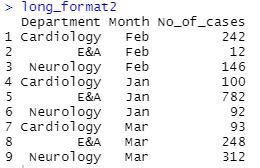top of page
Search
• Ekta Aggarwal

# Transposing / Reshaping data in R (wide and long formats)

In this tutorial we shall try to understand what are long and wide format datasets and how to convert them from wide to long and long to wide in R.

Key concepts:

### Long format:

Following is the example of a long data where both the attributes- department and month information are present as seperate columns and we have a different column for values.### Wide format:

In a wide format at least one of our attributes is present as multiple columns (here we have seperate columns for each month) and values are filled in these 2X2 table (where values are our number of cases corresponding to each department and month).Many a times we need to transpose our data i.e. switch from long or wide format or vice - versa.

## Using tidyr package:

```install.packages("tidyr")
library(tidyr)```

For transposing data using tidyr we shall use the following data:

```mydata  = data.frame(Department = c("Cardiology","Cardiology","Cardiology","E&A","E&A","E&A","Neurology","Neurology","Neurology"),
Month = c("Jan","Feb","Mar","Jan","Feb","Mar","Jan","Feb","Mar"),
No_of_cases = c(100,242,93,782,12,248,92,146,312))

print(mydata)```### Long to wide - Using spread function:

Syntax:

spread(data, key, value, fill = NA, convert = FALSE, drop = TRUE, sep = NULL)

data : Data to be transposed.

key = Variable which should contain the name of column in transposed dataset.

value = Variable where values are stored which shall be used for filling the transposed dataset.

fill = Missing values will be replaced by value mentioned in fill.

```wide_format = spread(mydata,Month,No_of_cases)
wide_format```### Wide to long - Using gather function:

Syntax:

gather(data, key, value, ..., na.rm = FALSE, convert = FALSE)

data : Data to be transposed.

key = Name of the new column which contains categories i.e. our key column in transposed data

value = Name of the new column which contains value i.e. our value column in transposed data

... = Vector of column name from where value should be taken

```long_format = gather(wide_format, key = "Month", value = "No_of_cases", Feb:Mar)
Alternatively
long_format2 = gather(wide_format,key = "Month",value = "No_of_cases",-Department)
long_format2```## Using data.table package:

```install.packages("data.table")
library(data.table)```

For transposing data using data.table we shall use the following data:

```mydata  = data.frame("Department" = c("Cardiology","Cardiology","Cardiology","E&A","E&A","E&A","Neurology","Neurology","Neurology"),
Month = c("Jan","Feb","Mar","Jan","Feb","Mar","Jan","Feb","Mar"),
No_of_cases = c(100,242,93,782,12,248,92,146,312),
No_of_deaths = c(10,4,11,54,19,29,32,43,22))

mydata = as.data.table(mydata)```

### Long to wide: Using dcast function

Syntax:

dcast( data, formula, fun.aggregate = NULL, sep = "_", ..., fill = NULL, value.var = guess(data))

data : Data to be transposed.

formula : LHS~RHS

(LHS :columns whose values are to be kept in rows in transposed data.

RHS: columns whose values are to be kept as new columns in transposed data

value.var = vector of column whose values have to be filled.

fill = Missing values will be replaced by value mentioned in fill.

`dcast(mydata,Department~Month,value.var = "No_of_cases")``dcast(mydata,Department~Month,value.var = "No_of_deaths")`Using dcast you can define multiple columns to be transposed!

`dcast(mydata,Department~Month, value.var = c("No_of_cases","No_of_deaths"))`### Wide to Long: Using melt function

Syntax:

melt( data, id.vars, variable.name = "variable", value.name = "value", ..., na.rm = FALSE)

data: Data to be transposed

id.vars : Name of columns which are our ids

variable.name : Name of the new column which contains categories i.e. our key column in transposed data

value.name : Name of the new column which contains value i.e. our value column in transposed data

```wide_format = as.data.table(wide_format)

melt(wide_format,id.vars = "Department",variable.name = "Month",value.name = "No_of_cases")```See All
bottom of page